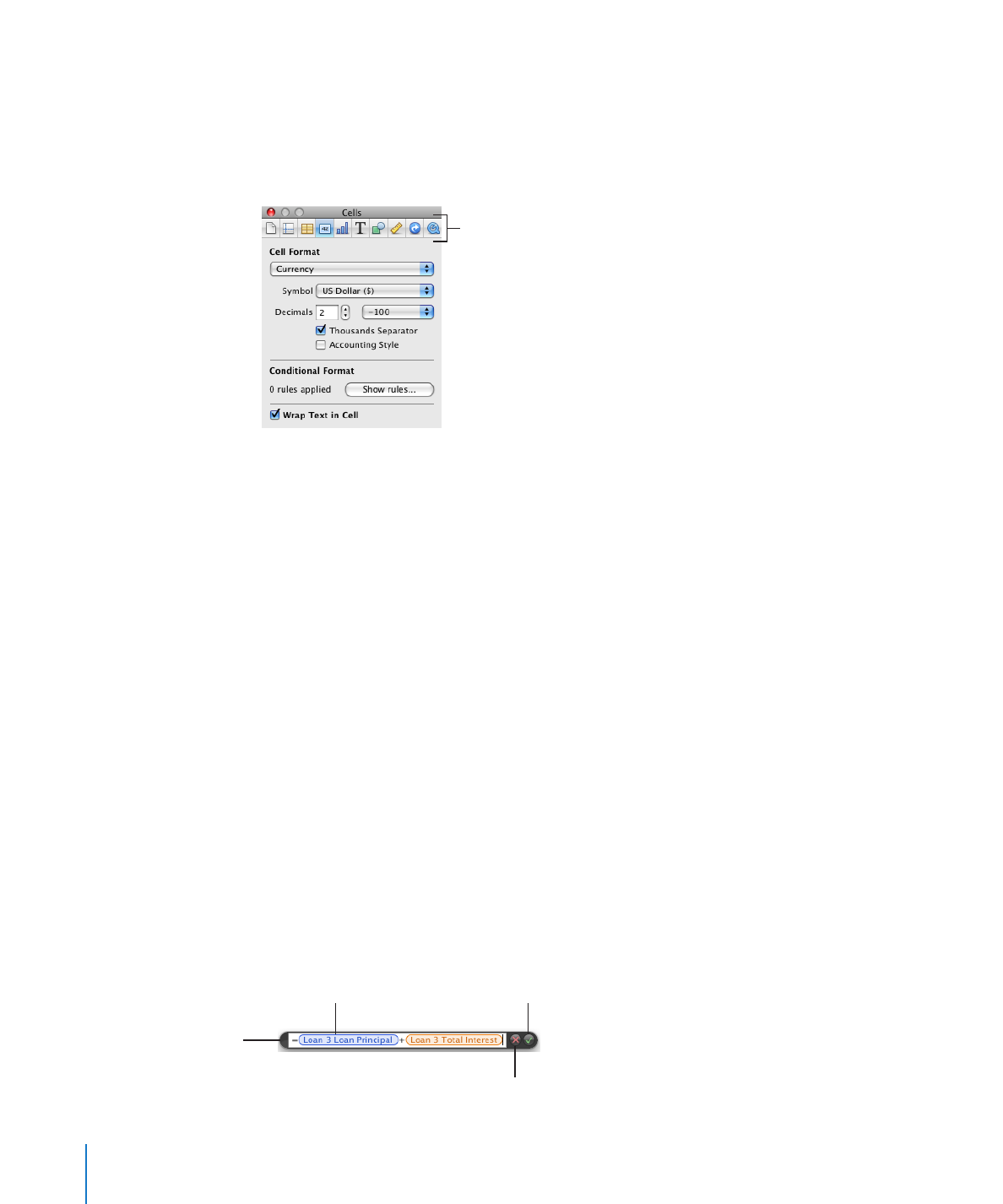## Numbers - Formula ToolsFormula Tools

You add a formula to a table cell when you want to display a value in the cell that’s
derived using a calculation. Numbers has several tools for working with formulas in
table cells:

The

Â

Formula Editor lets you create and modify formulas. Open the Formula Editor by

selecting a table cell and typing the equal sign (=). You can also open it by choosing
Formula Editor from the Function pop-up menu in the toolbar.

Cancel button

Accept button
Save changes.

Text field
View or edit a formula.

Formula Editor

Move by grabbing

here and dragging.

20

Chapter 1

Numbers Tools and TechniquesChapter 1

Numbers Tools and Techniques

21

Editor” on page 122.
The

Â

formula bar, always visible below the format bar, can also be used to create and

modify a formula in a selected table cell.

Open the
Function Browser.

Cancel button

Accept button
Save changes.

Change the formula
viewing size.

Text field
View or edit a formula.

Instructions for adding and editing formulas using this tool are in “Adding and
Editing Formulas Using the Formula Bar” on page 123.
Using the

Â

Function Browser is the fastest way to add a function. A function is a

predefined formula that has a name (such as SUM and AVERAGE).

Select a function to
view information

Search for a function.

Insert the selected function.

Select a category
to view functions in
that category.

To open the Function Browser, choose Show Function Browser from the Function